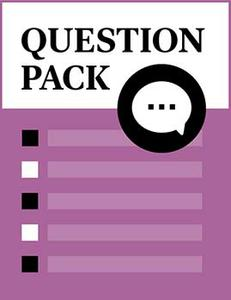# Dynamic System Application

Source: Saylor

Student Price: Free with Top Hat Pro Subscription

A question pack on dynamic systems and controls.

Dynamic System Applications Q17

Voltage in electrical systems is analogous to ______________ in fluid systems.

A

Flow

B

Pressure

C

Current

D

Torque

Dynamic System Applications Q19

How many types of electric power source are there in electrical systems?

A

Two

B

Three

C

Four

D

Five

Dynamic System Applications Q18

Which of the following are components of electrical systems?

A

Capacitor

B

Inductor

C

Resistor

D

All of the above

Dynamic System Applications Q21

Which of the following is an example of static dynamic system?

A

Gear train

B

C

Transformer

D

All of the above

Dynamic System Applications Q16

Which of the following is NOT a method of modeling electrical systems?

A

Loop method

B

Node method

C

Complex impedances

D

Perimeter method

Dynamic System Applications Q14

Bode’ plots are based on which of the following?

A

Time

B

Frequency

C

Both A and B

D

None of the above

Dynamic System Applications Q15

Decibels in Bode’ plot is used to measure which of the following?

A

Power gain

B

Phase

C

Margin

D

Percentage overshoot

Dynamic System Applications Q20

In Bode’ plot frequency is plotted in ______________.

A

Decibels

B

Logarithmic scale

C

Degrees

D

Percentage overshoot

Dynamic System Applications Q13

In Bode’ plot magnitude is plotted in ______________.

A

Decibels

B

Logarithmic scale

C

Degrees

D

Percentage overshoot

Dynamic System Applications Q12

In Bode’ plot phase is plotted in ______________.

A

Decibels

B

Logarithmic scale

C

Degrees

D

Percentage overshoot

Dynamic System Applications Q11

Which of the following about Nyquist plots is true?

A

A Nyquist plot uses the same plot to display amplitude and phase.

B

A Nyquist plot cannot depict if a system is stable or unstable.

C

Both A and B

D

None of the above

Dynamic System Applications Q10

In control systems, the device which measures what the system is doing is also known as which of the following?

A

Sensor

B

Input

C

Plant

D

None of the above

Dynamic System Applications Q9

In control systems, the system to be controlled is also known as which of the following?

A

Sensor

B

Input

C

Plant

D

None of the above

Dynamic System Applications Q8

Many heating systems use what type of control?

A

Proportional control

B

Integral control

C

On‑Off control

D

Derivative control

Dynamic System Applications Q7

The thermostat in homes is an example of which of the following?

A

Proportional

B

Integral

C

On‑Off

D

Derivative

Dynamic System Applications Q6

Digital control systems have been accelerated by the invention of ______________.

A

Vacuum tubes

B

Transformers

C

Microprocessors

D

Opamps

Dynamic System Applications Q4

Which of the following regarding digital control systems is false?

A

Digital control system uses digital computers.

B

Digital control system uses digital signals.

C

Measured data is converted from analog to digital.

D

Measured data is converted from digital to analog.

Dynamic System Applications Q5

Which of the following regarding manipulation of digital control is true?

A

Manipulation is done in digital form.

B

Manipulation is done in analog form.

C

Both A and B

D

None of the above

Dynamic System Applications Q3

Which of the following regarding many systems is true?

A

Many systems are analog.

B

Many systems are digital.

C

Both A and B

D

None of the above

Dynamic System Applications Q2

Which of the following regarding performance of linear digital systems is true?

A

Performance of analog is greater than performance of digital.

B

Performance of analog is less than performance of digital.

C

Performance of analog is equal to performance of digital.

D

None of the above

Dynamic System Applications Q1

Which of the following regarding performance of nonlinear digital systems is true?

A

Performance of analog is greater than performance of digital.

B

Performance of analog is less than performance of digital.

C

Performance of analog is equal to performance of digital.

D

None of the above.

CC BY 3.0 - Saylor